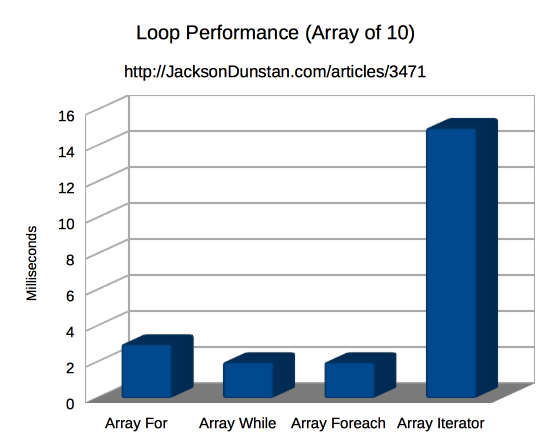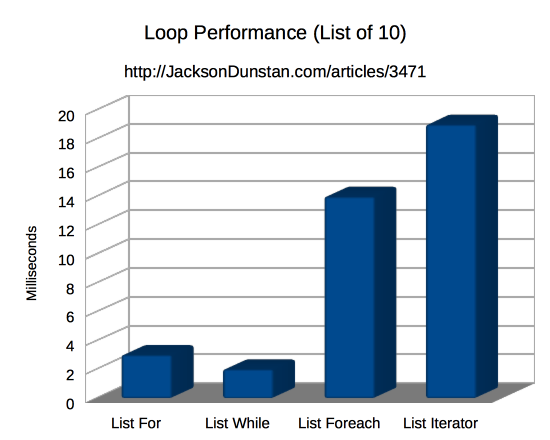In C#, just about everything is an `IEnumerable`. Since LINQ syntax, `foreach` loops, and the `System.Linq` namespace are all designed to work with `IEnumerable`, you’ve got lots of tools to use. Unfortunately, the core of `IEnumerable` is the `GetEnumerator` function which usually creates garbage and eventually causes memory fragmentation and GC framerate spikes. Do we simply stop using all of these nice tools? Normally the answer is “yes”, but today’s article shows you another way.

Today’s article is fundamentally about optimization. How do we optimize our code so that we don’t create any garbage while still having some tools to help us with the grunt work? It’s very handy to have tools like `foreach` loops and the extension methods in the `System.Linq` namespace, but they (almost always) end up creating garbage.

`IEnumerable` is really just an interface to provide the `GetEnumerator` function that gets you an `IEnumerator`. With an enumerator you can get the `Current` property, call `MoveNext` to go to the next element, `Reset` to move back to the start, and `Dispose` when you’re done.

This sort of enumerator is very general and can be used for any sequence of values. This includes arrays and `List` as well as other collection types (e.g. `Dictionary`) and iterator functions (i.e. those with `yield`). These all fit due to the generality of `IEnumerable`, but this also comes with a lack of power. For example, there’s no `MovePrev` function because you can’t go backwards in an iterator function.

The next tradeoff being made by `IEnumerable` is right in its name: it’s an interface. The interface automatically means that each function is `virtual` and can’t be implemented by a generic struct (i.e. `MyStruct<T>`) without the struct needing to be allocated on the heap as garbage for the GC to later collect. On the plus side, the interface allows for polymorphism to reduce code duplication. For example, the extension methods in `System.Linq` only needed to be implemented once and they work for any `IEnumerable`.

So how can we do it differently? Since we’re looking to create zero garbage for the GC then we need to rule out classes and interfaces. The natural alternative is `struct` which we can carefully arrange to never create any garbage. To avoid some confusion, let’s call our type an iterator rather than an enumerator. Here’s one for any kind of array:

```public struct ArrayIterator<T>
{
public T[] Array;
public int Index;
}```

Since this is a generic struct, we get to use it with arrays containing any type of elements. That’s crucial for code reuse, but unfortunately means that we can’t add any properties, methods, or non-default constructors. Instead, we can add some usability to this iterator type by adding extension methods onto it:

```public static class ArrayIteratorExtensions
{
public static ArrayIterator<T> Begin<T>(this T[] array)
{
return new ArrayIterator<T> { Array = array };
}

public static ArrayIterator<T> End<T>(this T[] array)
{
return new ArrayIterator<T> { Array = array, Index = array.Length };
}

public static ArrayIterator<T> IteratorAt<T>(this T[] array, int index)
{
return new ArrayIterator<T> { Array = array, Index = index };
}

public static T GetCurrent<T>(this ArrayIterator<T> it)
{
return it.Array[it.Index];
}

public static ArrayIterator<T> GetNext<T>(this ArrayIterator<T> it)
{
it.Index++;
return it;
}

public static ArrayIterator<T> GetPrev<T>(this ArrayIterator<T> it)
{
it.Index--;
return it;
}

public static bool IsEqual<T>(this ArrayIterator<T> it, ArrayIterator<T> other)
{
return it.Array == other.Array && it.Index == other.Index;
}

public static bool NotEqual<T>(this ArrayIterator<T> it, ArrayIterator<T> other)
{
return it.Array != other.Array || it.Index != other.Index;
}
}```

This is just a basic set, but already we have enough to perform common tasks such as looping. Here’s how that’d look:

```int FindMax(int[] array)
{
var max = int.MinValue;
for (
// Get iterators for the beginning and end
// Note: end is one past the last element
ArrayIterator<int> it = array.Begin(), end = array.End();
// Loop until the current iterator equals the end iterator
it.NotEqual(end);
// Move the current iterator forward
it = it.GetNext()
)
{
// Get the current iterator's current value
var cur = it.GetCurrent();
if (cur > max)
{
max = cur;
}
}
return max;
}```

Since using `List` and other list collections (e.g. `LinkedList`) is extremely common, let’s define a parallel set of iterator code for `IList`:

```public struct ListIterator<T>
{
public IList<T> List;
public int Index;
}

public static class ListIteratorExtensions
{
public static ListIterator<T> Begin<T>(this IList<T> List)
{
return new ListIterator<T> { List = List };
}

public static ListIterator<T> End<T>(this IList<T> List)
{
return new ListIterator<T> { List = List, Index = List.Count };
}

public static ListIterator<T> IteratorAt<T>(this IList<T> List, int index)
{
return new ListIterator<T> { List = List, Index = index };
}

public static T GetCurrent<T>(this ListIterator<T> it)
{
return it.List[it.Index];
}

public static ListIterator<T> GetNext<T>(this ListIterator<T> it)
{
it.Index++;
return it;
}

public static ListIterator<T> GetPrev<T>(this ListIterator<T> it)
{
it.Index--;
return it;
}

public static bool IsEqual<T>(this ListIterator<T> it, ListIterator<T> other)
{
return it.List == other.List && it.Index == other.Index;
}

public static bool NotEqual<T>(this ListIterator<T> it, ListIterator<T> other)
{
return it.List != other.List || it.Index != other.Index;
}
}```

Looping looks almost exactly the same with these:

```int FindMax(IList<int> list)
{
var max = int.MinValue;
for (
ListIterator<int> it = list.Begin(), end = list.End();
it.NotEqual(end);
it = it.GetNext()
)
{
var cur = it.GetCurrent();
if (cur > max)
{
max = cur;
}
}
return max;
}```

Again, this is just a very basic set of iterator functions but it does provide a foundation to build on. For a glimpse of what can be built, see the documentation for the `algorithm` header in C++.

Finally, let’s look at the performance of these basic loops against `for`, `while`, and `foreach` with arrays and `List`. I’ve simply added on an iterator-based loop to the tests from this recent article to come up with this little test script:

```using System;
using System.Collections.Generic;
using UnityEngine;

public class TestScript : MonoBehaviour
{
private const int NumIterations = 100000;

private class Counter
{
public int Value;
}

private string report;

void Start()
{
report = string.Join(
",",
new[]{
"Size",
"Array For",
"Array While",
"Array Foreach",
"Array Iterator",
"List For",
"List While",
"List Foreach",
"List Iterator"
}
) + "\n";
var sizes = new []{ 10,100,1000 };
foreach (var size in sizes)
{
report += Test(size);
}
}

private string Test(int size)
{
var stopwatch = new System.Diagnostics.Stopwatch();
var sum = 0;
var array = new Counter[size];
for (int i = 0; i < size; ++i)
{
array[i] = new Counter() { Value = i };
}

stopwatch.Reset();
stopwatch.Start();
for (var iteration = 0; iteration < NumIterations; ++iteration)
{
for (int i = 0, len = array.Length; i < len; ++i)
{
sum += array[i].Value;
}
}
var arrayForTime = stopwatch.ElapsedMilliseconds;

stopwatch.Reset();
stopwatch.Start();
for (var iteration = 0; iteration < NumIterations; ++iteration)
{
var i = 0;
var len = array.Length;
while (i < len)
{
sum += array[i].Value;
++i;
}
}
var arrayWhileTime = stopwatch.ElapsedMilliseconds;

stopwatch.Reset();
stopwatch.Start();
for (var iteration = 0; iteration < NumIterations; ++iteration)
{
foreach (var cur in array)
{
sum += cur.Value;
}
}
var arrayForeachTime = stopwatch.ElapsedMilliseconds;

stopwatch.Reset();
stopwatch.Start();
for (var iteration = 0; iteration < NumIterations; ++iteration)
{
for (
ArrayIterator<Counter> it = array.Begin(), end = array.End();
it.NotEqual(end);
it = it.GetNext()
)
{
sum += it.GetCurrent().Value;
}
}
var arrayIteratorTime = stopwatch.ElapsedMilliseconds;

var list = new List<Counter>(size);

stopwatch.Reset();
stopwatch.Start();
for (var iteration = 0; iteration < NumIterations; ++iteration)
{
for (int i = 0, len = list.Count; i < len; ++i)
{
sum += list[i].Value;
}
}
var listForTime = stopwatch.ElapsedMilliseconds;

stopwatch.Reset();
stopwatch.Start();
for (var iteration = 0; iteration < NumIterations; ++iteration)
{
var i = 0;
var len = list.Count;
while (i < len)
{
sum += list[i].Value;
++i;
}
}
var listWhileTime = stopwatch.ElapsedMilliseconds;

stopwatch.Reset();
stopwatch.Start();
for (var iteration = 0; iteration < NumIterations; ++iteration)
{
foreach (var cur in list)
{
sum += cur.Value;
}
}
var listForeachTime = stopwatch.ElapsedMilliseconds;

stopwatch.Reset();
stopwatch.Start();
for (var iteration = 0; iteration < NumIterations; ++iteration)
{
for (
ListIterator<Counter> it = list.Begin(), end = list.End();
it.NotEqual(end);
it = it.GetNext()
)
{
sum += it.GetCurrent().Value;
}
}
var listIteratorTime = stopwatch.ElapsedMilliseconds;

return size + ","
+ arrayForTime + ","
+ arrayWhileTime + ","
+ arrayForeachTime + ","
+ arrayIteratorTime + ","
+ listForTime + ","
+ listWhileTime + ","
+ listForeachTime + ","
+ listIteratorTime + "\n";
}

void OnGUI()
{
GUI.TextArea(new Rect(0, 0, Screen.width, Screen.height), report);
}
}```

If you want to try out the test yourself, simply paste the above code into a `TestScript.cs` file in your Unity project’s `Assets` directory and attach it to the main camera game object in a new, empty project. Then build in non-development mode for 64-bit processors and run it windowed at 640×480 with fastest graphics. I ran it that way on this machine:

• 2.3 Ghz Intel Core i7-3615QM
• Mac OS X 10.11.5
• Unity 5.3.4f1, Mac OS X Standalone, x86_64, non-development
• 640×480, Fastest, Windowed

And here are the results I got:

Size Array For Array While Array Foreach Array Iterator List For List While List Foreach List Iterator
10 3 2 2 15 3 2 14 19
100 28 29 27 151 36 31 89 170
1000 259 267 289 1497 339 294 831 1739The performance of the iterator-based loops is clearly worse than any alternative. This is especially true in the case of arrays where the performance penalty is roughly 7x. With `List`, however, the penalty is about the same compared to `for` and `while` but only about 2x slower than `foreach`.

So is this iterator-based looping unusably slow? Not necessarily. There are two very important factors to keep in mind while interpreting these results. First, remember that all types of these loops were executed in very little time. Each array or list was iterated over 100,000 times to get a big enough number to show up in the results. Even with the slowest type (`List`) the iterator-based loop was performing over 50,000 iterations per millisecond! Even this is artificially high since it counts the work to add to the `sum` variable and the overhead of the outer loop. In reality you’d get even more iterations per second.

The second important factor to keep in mind is that the iterator-based loops didn’t create any garbage. The `foreach` loop on the `List` created almost 4 MB! (40 bytes * 100,000 loops) The performance penalty for all this garbage creation isn’t paid up front when the garbage is created but later on down the line after the test has been completed and the GC needs to collect it all. If you add that cost onto `foreach` for `List` then it will likely be much slower than the iterator-based loop and much more unpredictable and uncontrollable since the GC runs whenever Unity decides it should.

That’s all for part one of this series. In future articles I’ll be adding on to the basic set of iterator extension functions to create a useful little library. It should serve as an alternative to `IEnumerable` for those of us concerned with garbage creation. Stay tuned!

UPDATE: check out part two